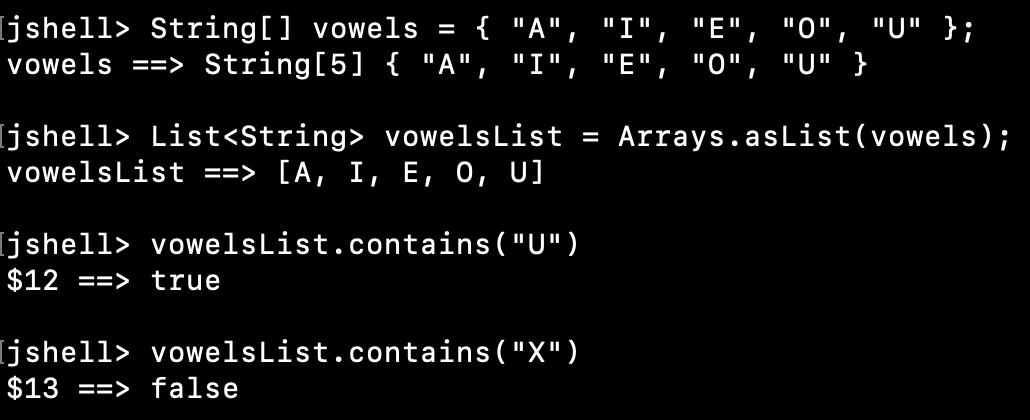# How to Check if Java Array Contains a Value?

Published on August 3, 2022By Pankaj
Developer and author at DigitalOcean.While we believe that this content benefits our community, we have not yet thoroughly reviewed it. If you have any suggestions for improvements, please let us know by clicking the “report an issue“ button at the bottom of the tutorial.

## How to Check if Java Array Contains a Value?

There are many ways to check if a Java array contains a specific value.

• Simple iteration using for loop
• List contains() method
• Stream anyMatch() method
• Arrays binarySearch() for sorted array

Let’s look into all these methods one at a time.

### 1. Using For Loop

This is the easiest and convenient method to check if the array contains a certain value or not. We will go over the array elements using the for loop and use the equals() method to check if the array element is equal to the given value.

``````String[] vowels = { "A", "I", "E", "O", "U" };

// using simple iteration over the array elements
for (String s : vowels) {
if ("E".equals(s)) {
System.out.println("E found in the vowels list.");
}
}
``````

### 2. Using List contains() method

We can use Arrays class to get the list representation of the array. Then use the contains() method to check if the array contains the value. Let’s use JShell to run the example code snippet.

``````jshell> String[] vowels = { "A", "I", "E", "O", "U" };
vowels ==> String { "A", "I", "E", "O", "U" }

jshell> List vowelsList = Arrays.asList(vowels);
vowelsList ==> [A, I, E, O, U]

jshell> vowelsList.contains("U")
\$3 ==> true

jshell> vowelsList.contains("X")
\$4 ==> false
``````### 3. Using Stream anyMatch() Method

If you are using Java 8 or higher, you can create a stream from the array. Then use the anyMatch() method with a lambda expression to check if it contains a given value.

``````jshell> List vowelsList = Arrays.asList(vowels);
vowelsList ==> [A, I, E, O, U]

jshell> Arrays.stream(vowels).anyMatch("O"::equals);
\$5 ==> true

jshell> Arrays.stream(vowels).anyMatch("X"::equals);
\$6 ==> false
``````

### 4. Arrays binarySearch() for sorted array

If your array is sorted, you can use the Arrays binarySearch() method to check if the array contains the given value or not.

``````String[] vowels = { "A", "I", "E", "O", "U" };

System.out.println("Unsorted Array = " + Arrays.toString(vowels));

Arrays.parallelSort(vowels);

System.out.println("Sorted Array = " + Arrays.toString(vowels));

int index = Arrays.binarySearch(vowels, "X");

if (index < 0) {
} else {
System.out.println("X found in the array");
}
``````

Output:

``````Unsorted Array = [A, I, E, O, U]
Sorted Array = [A, E, I, O, U]
``````

## Checking if Array Contains Multiple Values

What if we want to check if the array contains multiple values. Let’s say you want to check if a given array is the subset of the source array. We can create nested loops and check each element one by one. There is a cleaner way by converting arrays to list and then use the containsAll() method.

``````String[] vowels = { "A", "I", "E", "O", "U" };
String[] subset = { "E", "U" };

boolean foundAll = Arrays.asList(vowels).containsAll(Arrays.asList(subset));

System.out.println("vowels contains all the elements in subset = " + foundAll);
``````

Output: vowels contains all the elements in subset = true